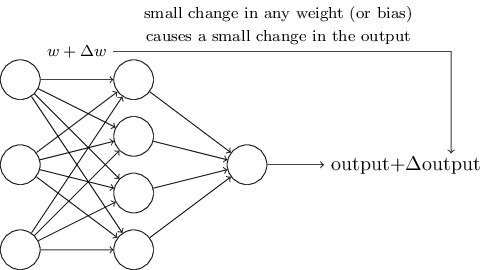阿扣：上一次我们了解了损失函数。为了找到使损失函数（比如用 SSE 计算）最小的 w (权重) 和 b (偏置项)，我们需要先了解一个重要的方法：梯度下降。

阿特：听起来像坐滑滑梯~

阿扣：是有那么点意思。

阿扣：想象一下，我们对网络中的一些权重做了很小的改变，这些变化会让输出也有相应很小的变化：阿特：那要怎么找到这些值呢？

阿扣：下面有请「梯度下降」 Gradient Descent。

阿特：终于能坐滑滑梯了……

阿扣：坐这个滑滑梯可能有点晕 😄 。我先给你打个比方。想象一下，你在一个山峰的山顶，想用最快的速度到达山脚。

阿特：坐缆车可以吗？

阿扣：缆车，不存在的……只能靠走的。要往哪边下山呢？我们会选一个看起来「下降」最快的路径：阿特：是这么个道理。

阿扣：这个方法，就是「梯度下降」，在机器学习中很常见。所谓「梯度」，其实是指「变化率」或者「坡度 slope」，就是多变量函数的导数。

阿特：导数？！你说的是微积分里面那个导数吗？ …… 瑟瑟发抖.gif

阿扣：别紧张，先听我讲，回忆回忆。

阿特：好吧。

阿扣：你还记得怎么表示函数 f(x) 的导数吧？很简单，就是 f’(x) 。

阿特：嗯嗯，记得。

阿扣：所谓「梯度」，其实就是函数在某一点上的变化率，根据微分的知识，变化率可以通过这一点的切线求得，而切线其实就是函数的导数：f’(x)。阿特：求梯度 = 求变化率 = 求导数 （假装自己听懂了）

阿扣：了解了「梯度」，然后我们来看看「下降」又是怎么回事。

阿特：沿着「上山」最快的反方向走，就能最快「下山」。啊原来这么直白……

阿扣：对呀，原理并不复杂的。

阿特：这个视频不错，讲得挺清楚的~

阿扣：我们来复习一下。用一个函数 f(h) 表示 x 和 y 的关系。x 和 y 其实是已知的，它们来自真实的数据集。我们的目标是求出 w 和 b，使得计算出来的 $\hat y$ 最接近实际的 y 值。为了得到某种类型的 y 值（比如只有 0 和 1 两种输出），我们会使用类似 Sigmoid 这样的激活函数，对 f(h) 做一下转换。阿特：哦，我说怎么有点难理解呢。因为以前碰到 x 和 y，它们都是未知数，现在它们变成了已知数，真正的目标其实是求 w 和 b！

阿扣：没错！这是深度学习算法中一个需要调整的认知。阿扣：比如说，如果激活函数是 Sigmoid 函数。

$$f(h)=\frac {1}{1 + e^{−h}}$$

$$f’(h)=f(h)(1−f(h))$$

$$\Delta w_{ij}=\eta(y_j-\hat y_j)f(h)(1−f(h))x_i$$

…… 咦？人呢？

### 补充：求多个变量的偏导数

$$\frac{\partial}{\partial w} p(q(w)) = \frac{\partial p}{\partial q}\frac{\partial q}{\partial w}$$

$$C = \sum(wx + b - y)^2 = \sum((wx + b)^2 + y^2 - 2y(wx + b)) = \sum(x^2w^2 + b^2 + 2xwb + y^2 - 2xyw - 2yb)$$

$$\frac{\partial C}{\partial w} = \frac{1}{N} \sum(wx + b - y)x$$

$$\frac{\partial C}{\partial b} = \frac{1}{N} \sum(wx + b - y)$$

### 00 的 DeepLearning 笔记